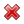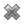# Fight Finance

#### CoursesTagsRandomAllRecentScoresScoreskeithphw $6,011.61 Jade$1,815.80 Chu $789.98 royal ne...$750.00 Leehy $713.33 ZOE HY$660.00 Visitor $650.00 JennyLI$625.61 Visitor $590.00 Visitor$555.33 Visitor $550.00 Visitor$550.00 Visitor $540.00 Visitor$500.00 Yizhou $489.18 Visitor$480.00 Visitor $480.00 Visitor$470.00 Visitor $464.70 LWH$460.00

A company has:

• 50 million shares outstanding.
• The market price of one share is currently $6. • The risk-free rate is 5% and the market return is 10%. • Market analysts believe that the company's ordinary shares have a beta of 2. • The company has 1 million preferred stock which have a face (or par) value of$100 and pay a constant dividend of 10% of par. They currently trade for $80 each. • The company's debentures are publicly traded and their market price is equal to 90% of their face value. • The debentures have a total face value of$60,000,000 and the current yield to maturity of corporate debentures is 10% per annum. The corporate tax rate is 30%.

What is the company's after-tax weighted average cost of capital (WACC)? Assume a classical tax system.

Which one of the following bonds is trading at par?

One formula for calculating a levered firm's free cash flow (FFCF, or CFFA) is to use earnings before interest and tax (EBIT).

\begin{aligned} FFCF &= (EBIT)(1-t_c) + Depr - CapEx -\Delta NWC + IntExp.t_c \\ &= (Rev - COGS - Depr - FC)(1-t_c) + Depr - CapEx -\Delta NWC + IntExp.t_c \\ \end{aligned} \\
Does this annual FFCF or the annual interest tax shield?

A levered company's required return on debt is always less than its required return on equity. or ?

What is the lowest and highest expected share price and expected return from owning shares in a company over a finite period of time?

Let the current share price be $p_0$, the expected future share price be $p_1$, the expected future dividend be $d_1$ and the expected return be $r$. Define the expected return as:

$r=\dfrac{p_1-p_0+d_1}{p_0}$

The answer choices are stated using inequalities. As an example, the first answer choice "(a) $0≤p<∞$ and $0≤r< 1$", states that the share price must be larger than or equal to zero and less than positive infinity, and that the return must be larger than or equal to zero and less than one.

If a stock's future expected future prices are log-normally distributed, what will be bigger, the stock's or future price? Or would you expect them to be ?

Which of the following terms about options are NOT synonyms?

Question 841  gross domestic product, government spending, no explanation

The government spends money on:

• Goods and services such as defence, police, schools, hospitals and roads; and
• Transfer payments (also called welfare) such as the pension, dole, disability support and student support.

When calculating GDP, the ‘government spending’ component is supposed to include:

If the Australian dollar quote of 0.8 USD per AUD suddenly falls to 0.7 USD per AUD, has the Australian dollar or against the US dollar?

Question 906  effective rate, return types, net discrete return, return distribution, price gains and returns over time

For an asset's price to double from say $1 to$2 in one year, what must its effective annual return be? Note that an effective annual return is also called a net discrete return per annum. If the price now is $P_0$ and the price in one year is $P_1$ then the effective annul return over the next year is:

$$r_\text{effective annual} = \dfrac{P_1 - P_0}{P_0} = \text{NDR}_\text{annual}$$